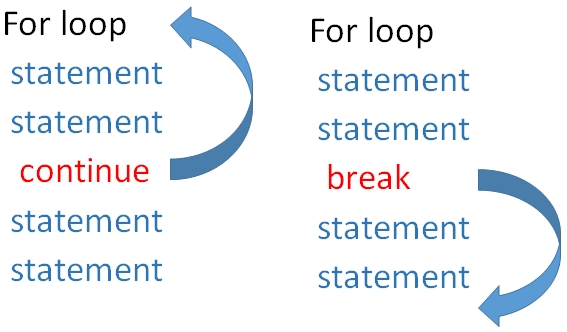# For Loop in Python

For loop with range
``````for x in range(5):
print(x)``````
Note that the 2nd line print(x) is placed after an indente of one space. Without that we will get an error asking expectd an indented block . The code to be executed within the block is to be kept with indenting as there is no end of for loop like other languages.
The output of above code is here
``````0
1
2
3
4``````
Here is a statement which is outside the loop because there is no indenting at the beginning of the line
``````for x in range(5):
print(x)
print("I am outside the loop")
``````
Output is here .
``````0
1
2
3
4
I am outside the loop``````
In the above code the range is from 0 to 5 ( excluding 5 ) , so we get output from 0 to 4.

## Loop with range

Now we will define a range for the loop
``````for x in range(5,10):
print(x)``````
Output is here
``````5
6
7
8
9``````
We can add a increment value
``````for x in range(5,25,5):
print(x)``````
Output is here
``````5
10
15
20``````

## Break and continue in a for loop

When break statement is encountered the execution comes out of the loop. In case of continue the execution returns to the starting of the loop skipping the rest of the statements ( after continue ) and continues again.

In the codes give below , as soon as break is encountered the execution comes out of the loop, so 15 and 20 are not printed. But when continue is used in place of break the printing of 15 is skipped but the loop continues again so 20 is printed.Using continue
``````for x in range(5,25,5):
if(x==15):
continue
print(x)``````
``````5
10
20``````
Using break
``````for x in range(5,25,5):
if(x==15):
break
print(x)``````
``````5
10``````

## Using else with for loop

Here else part of the code is executed once the for loop execution is over. However if loop is terminated by using break then else part of the code is skipped.

Using continue
``````for x in range(5,25,5):
if(x==15):
continue
print(x)
else:
print("Out of loop but with else")
print("Out of loop")``````
Output is here
``````5
10
20
Out of loop but with else
Out of loop``````
using break
``````for x in range(5,25,5):
if(x==15):
break
print(x)
else:
print("Out of loop but with else")
print("Out of loop")``````
Output is here
``````5
10
Out of loop``````

## Using string

``````names=["King","Queen","Jack & others"]
for str in names:
print(str)``````
Output is here
``````King
Queen
Jack & others``````

## Nested for loop

We can keep one for loop inside another
``````names=["King","Queen","Jack & others"]
ages=["child","Young","old"]
for str in names:
for str2 in ages:
print(str,str2)``````
Output is here
``````King child
King Young
King old
Queen child
Queen Young
Queen old
Jack & others child
Jack & others Young
Jack & others old``````
Your Rating

Post your comments , suggestion , error , requirements etc here

 HTML MySQL PHP JavaScript ASP Photoshop Articles FORUM . Contact us
©2000-2019 plus2net.com All rights reserved worldwide Privacy Policy Disclaimer